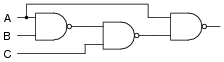# Boolean Algebra

## Digital Circuits

• #### Question 1

 Don’t just sit there! Build something!!

Learning to analyze digital circuits requires much study and practice. Typically, students practice by working through lots of sample problems and checking their answers against those provided by the textbook or the instructor. While this is good, there is a much better way.

You will learn much more by actually building and analyzing real circuits, letting your test equipment provide the “answers” instead of a book or another person. For successful circuit-building exercises, follow these steps:

1. Draw the schematic diagram for the digital circuit to be analyzed.
2. Carefully build this circuit on a breadboard or other convenient medium.
3. Check the accuracy of the circuit’s construction, following each wire to each connection point, and verifying these elements one-by-one on the diagram.
4. Analyze the circuit, determining all output logic states for given input conditions.
5. Carefully measure those logic states, to verify the accuracy of your analysis.
6. If there are any errors, carefully check your circuit’s construction against the diagram, then carefully re-analyze the circuit and re-measure.

Always be sure that the power supply voltage levels are within specification for the logic circuits you plan to use. If TTL, the power supply must be a 5-volt regulated supply, adjusted to a value as close to 5.0 volts DC as possible.

One way you can save time and reduce the possibility of error is to begin with a very simple circuit and incrementally add components to increase its complexity after each analysis, rather than building a whole new circuit for each practice problem. Another time-saving technique is to re-use the same components in a variety of different circuit configurations. This way, you won’t have to measure any component’s value more than once.

• #### Question 2

Identify each of these logic gates by name, and complete their respective truth tables:• #### Question 3

Identify each of these relay logic functions by name (AND, OR, NOR, etc.) and complete their respective truth tables:• #### Question 4

The following set of mathematical expressions is the complete set of “times tables” for the Boolean number system:

$$0 × 0 = 0$$

$$0 \ x \ 1=0$$

$$1 \ x \ 0=0$$

$$1 \ x \ 1=1$$

Now, nothing seems unusual at first about this table of expressions, since they appear to be the same as multiplication understood in our normal, everyday system of numbers. However, what is unusual is that these four statements comprise the entire set of rules for Boolean multiplication!

Explain how this can be so, being that there is no statement saying 1 ×2 = 2 or 2 ×3 = 6. Where are all the other numbers besides 0 and 1?

• #### Question 5

Boolean algebra is a strange sort of math. For example, the complete set of rules for Boolean addition is as follows:

$$0+0=0$$

$$0+1=1$$

$$1+0=1$$

$$1+1=1$$

Suppose a student saw this for the very first time, and was quite puzzled by it. What would you say to him or her as an explanation for this? How in the world can 1 + 1 = 1 and not 2? And why are there no more rules for Boolean addition? Where is the rule for 1 + 2 or 2 + 2?

• #### Question 6

Surveying the rules for Boolean addition, the 0 and 1 values seem to resemble the truth table of a very common logic gate. Which type of gate is this, and what does this suggest about the relationship between Boolean addition and logic circuits?

$$0+0=0$$
$$0+1=1$$
$$1+0=1$$
$$1+1=1$$

• #### Question 7

Surveying the rules for Boolean multiplication, the 0 and 1 values seem to resemble the truth table of a very common logic gate. Which type of gate is this, and what does this suggest about the relationship between Boolean multiplication and logic circuits?

 Rules for Boolean multiplication:

$$0 \ x \ 0=0$$
$$0 \ x \ 1=0$$
$$1 \ x \ 0=0$$
$$1 \ x \ 1 =1$$

• #### Question 8

What is the complement of a Boolean number? How do we represent the complement of a Boolean variable, and what logic circuit function performs the complementation function?

• #### Question 9

There are three fundamental operations in Boolean algebra: addition, multiplication, and inversion. Each of these operations has an equivalent logic gate function and an equivalent relay circuit configuration. Draw the corresponding gate and ladder logic diagrams for each:• #### Question 10

Write the Boolean expression for each of these logic gates, showing how the output (Q) algebraically relates to the inputs (A and B):• #### Question 11

Write the Boolean expression for each of these relay logic circuits, showing how the output (Q) algebraically relates to the inputs (A and B):• #### Question 12

Convert the following logic gate circuit into a Boolean expression, writing Boolean sub-expressions next to each gate output in the diagram: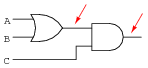• #### Question 13

Convert the following logic gate circuit into a Boolean expression, writing Boolean sub-expressions next to each gate output in the diagram: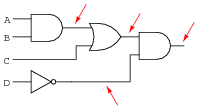• #### Question 14

Convert the following logic gate circuit into a Boolean expression, writing Boolean sub-expressions next to each gate output in the diagram: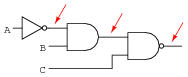• #### Question 15

Convert the following relay logic circuit into a Boolean expression, writing Boolean sub-expressions next to each relay coil and lamp in the diagram:• #### Question 16

Convert the following relay logic circuit into a Boolean expression, writing Boolean sub-expressions next to each relay coil and lamp in the diagram:• #### Question 17

Convert the following relay logic circuit into a Boolean expression, writing Boolean sub-expressions next to each relay coil and lamp in the diagram:• #### Question 18

An automotive engineer wants to design a logic circuit that prohibits the engine in a car from being started unless the driver is pressing the clutch pedal while turning the ignition switch to the “start” position. The purpose of this feature will be to prevent the car from moving forward while being started if ever the transmission is accidentally left in gear.

Suppose we designate the status of the ignition switch “start” position with the Boolean variable S (1 = start; 0 = run or off), and the clutch pedal position with the Boolean variable C (1 = clutch pedal depressed; 0 = clutch pedal in normal, unpressed position). Write a Boolean expression for the starter solenoid status, given the start switch (S) and clutch (C) statuses. Then, draw a logic gate circuit to implement this Boolean function.

• #### Question 19

An engineer hands you a piece of paper with the following Boolean expression on it, and tells you to build a gate circuit to perform that function:

$$A\overline{B}+\overline{C}(A+B)$$

Draw a logic gate circuit for this function.

• #### Question 20

A critical electronic system receives DC power from three power supplies, each one feeding through a diode, so that if one power supply develops an internal short-circuit, it will not cause the others to overload: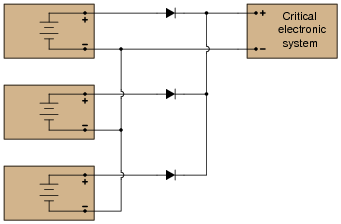The only problem with this system is that we have no indication of trouble if just one or two power supplies do fail. Since the diode system routes power from any available supply(ies) to the critical system, the system sees no interruption in power if one or even two of the power supplies stop outputting voltage. It would be nice if we had some sort of alarm system installed to alert the technicians of a problem with any of the power supplies, long before the critical system was in jeopardy of losing power completely.

An engineer decides that a relay could be installed at the output of each power supply, prior to the diodes. Contacts from these relays could then be connected to some sort of alarm device (flashing light, bell, etc.) to alert maintenance personnel of any problem: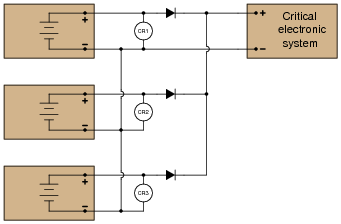Part 1: Draw a ladder diagram of the relay contacts powering a warning lamp, in such a way that the lamp energizes if any one or more of the power supplies loses output voltage. Write the corresponding Boolean expression for this circuit, using the letters A, B, and C to represent the status of relay coils CR1, CR2, and CR3, respectively.

Part 2: The solution to Part 1 worked, but unfortunately it generated “nuisance alarms” whenever a technician powered any one of the supplies down for routine maintenance. The engineer decides that a two-out-of-three-failed alarm system will be sufficient to warn of trouble, while allowing for routine maintenance without creating unnecessary alarms. Draw a ladder diagram of the relay contacts powering a warning lamp, such that the lamp energizes if any two or more power supplies lose output voltage. The Boolean expression for this is $$\overline{A} \ \overline{B}+\overline{B} \ \overline{C} + \overline{A} \ \overline{C}$$.

Part 3: Management at this facility changed their minds regarding the safety of a two-out-of-three-failed alarm system. They want the alarm to energize if any one of the power supplies fails. However, they also realize that nuisance alarms generated during routine maintenance are unacceptable as well. Asking the maintenance crew to come up with a solution, one of the technicians suggests inserting a “maintenance” switch that will disable the alarm during periods of maintenance, allowing for any of the power supplies to be powered down without creating a nuisance alarm. Modify the alarm circuit of part 1’s solution to include such a switch, and correspondingly modify the Boolean expression for the new circuit (call the maintenance switch M).

Part 4: During one maintenance cycle, a technician accidentally left the alarm bypass switch (M) actuated after he was done. The system operated with the power failure alarm disabled for weeks. When management discovered this, they were furious. Their next suggestion was to have the bypass switch change the conditions for alarm, such that actuating this “M” switch would turn the system from a one-out-of-three-failed alarm into a two-out-of-three-failed alarm. This way, any one power supply may be taken out of service for routine maintenance, yet the alarm will not be completely de-activated. The system will still alarm if two power supplies were to fail. The simplified Boolean expression for this rather complex function is $$\overline{A} \ \overline{B}+\overline{C} \ \overline{M} + (\overline{A} \ +\overline{B})+ (\overline{C} \ +\overline{M})$$. Draw a ladder diagram for the alarm circuit based on this expression.

• #### Question 21

Implement the following Boolean expression in the form of a digital logic circuit:

$$\overline{(\overline{AB}+C)}B$$

Form the circuit by making the necessary connections between pins of these integrated circuits on a solderless breadboard: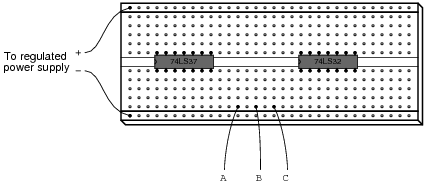• #### Question 22

Complete the truth tables for these two Boolean expressions:

$$Output = \overline{A}+B$$

 A B Output 0 0 0 1 1 0 1 1

$$Output =A+\overline{A}B$$

 A B Output 0 0 0 1 1 0 1 1

• #### Question 23

Complete the truth tables for these two Boolean expressions:
$$Output =\overline{A}+\overline{B}+C$$

 A B C Output 0 0 0 0 0 1 0 1 0 0 1 1 1 0 0 1 0 1 1 1 0 1 1 1

$$Output =A(B+AC+\overline{A})$$

 A B C Output 0 0 0 0 0 1 0 1 0 0 1 1 1 0 0 1 0 1 1 1 0 1 1 1

• #### Question 24

Like real-number algebra, Boolean algebra is subject to the laws of commutation, association, and distribution. These laws allow us to build different logic circuits that perform the same logic function.

For each of the equivalent circuit pairs shown, write the corresponding Boolean law next to it:Note: the three short, parallel lines represent ëquivalent to” in mathematics.

• #### Question 25

Like real-number algebra, Boolean algebra is subject to certain rules which may be applied in the task of simplifying (reducing) expressions. By being able to algebraically reduce Boolean expressions, it allows us to build equivalent logic circuits using fewer components.

For each of the equivalent circuit pairs shown, write the corresponding Boolean rule next to it:Note: the three short, parallel lines represent “equivalent to” in mathematics.

• #### Question 26

Shown here are six rules of Boolean algebra (these are not the only rules, of course).

$$A+\overline{A} = 1$$
$$A+A = A$$
$$A+1 = 1$$
$$AA = A$$
$$A+AB = A$$
$$A+ \overline{A}B = A+B$$

Determine which rule (or rules) are being used in the following Boolean reductions:

$$\overline{DF}+\overline{DF}C=\overline{DF}$$

$$1+G = 1$$

$$B+AB = B$$

$$\overline{FE}+\overline{FE}=\overline{FE}$$

$$XYZ+\overline{XYZ}=1$$

$$GQ+Q = Q$$

$$\overline{H} \ \overline{H}=\overline{H}$$

$$\overline{CD} + \overline{CD}=\overline{CD}$$

$$EF(EF) = EF$$

$$CD+\overline{C}=\overline{C}+D$$

$$LNM+ML = LM$$

$$A\overline{G}F\overline{C}+F\overline{C}\ \overline{G}=F\overline{C}\ \overline{G}$$

$$\overline{M}+1=1$$

$$\overline{BC}+BC=1$$

$$ABC+CAB = BCA$$

$$S+STV\overline{Q}=S$$

$$\overline{DE}(R+1)=\overline{DE}$$

$$\overline{RS}\ \overline{SR}=\overline{RS}$$

$$ABC\overline{D}+D=D+ABC$$

$$AC\overline{B}+CAD\overline{B}=A\overline{B}C$$

$$A+T+\overline{W}+\overline{A}+X=1$$

$$X\overline{YZ}+\overline{X}=\overline{X}+\overline{YZ}$$

$$\overline{GFH} \ \overline{HGF}=\overline{FHG}$$

$$C\overline{AB}+AB=AB+C$$

• #### Question 27

Shown here are eight rules of Boolean algebra (these are not the only rules, of course).

$$A+\overline{A}=1$$
$$A+A = A$$
$$A+1 = 1$$
$$AA = A$$
$$A\overline{A}=0$$
$$A(B++C) = AB+AC$$
$$A+AB = A$$
$$A+\overline{A}B=A+B$$

Determine which rule is being used in each step of the following Boolean simplification:

$$AB+B(B+\overline{C})+\overline{B}C$$

$$AB+BB+B\overline{C}+\overline{B}C$$

$$AB+B+B\overline{C}+\overline{B}C$$

$$AB+B+B\overline{C}$$

$$AB+B+C$$

$$B+C$$

• #### Question 28

A student makes a mistake somewhere in the process of simplifying the following Boolean expression:

$$AB + A(B + C)$$
$$AB + AB + C$$
$$AB + C$$

Determine where the mistake was made, and what the proper sequence of steps should be to simplify the original expression.

• #### Question 29

Factoring is a powerful simplification technique in Boolean algebra, just as it is in real-number algebra. Show how you can use factoring to help simplify the following Boolean expressions:

$$C + CD$$

$$A\overline{B}C + A\overline{B}\ \overline{C}$$

$$XY\overline{Z} + XYZ + XYW$$

$$\overline{D}EF + AB + \overline{D}E + 0 + ABC$$

• #### Question 30

Shown here are nine rules of Boolean algebra (these are not the only rules, of course).

$$A + \overline{A} = 1$$
$$A + A = A$$
$$A + 1 = 1$$
$$AA = A$$
$$A(1) = A$$
$$A \overline{A} = 0$$
$$A(B + C) = AB + AC$$
$$A + AB = A$$
$$A \overline{A}B = A+B$$

Determine which rule is being used in each step of the following Boolean simplification:

$$\overline{C}F + F(A+\overline{B})+C$$

$$\overline{C}F + AF + \overline{B}F + C$$

$$C + F + AF + \overline{B}F$$

$$C + F(1+A+\overline{B})$$

$$C + F(1)$$

$$C + F$$

• #### Question 31

Two very important rules of simplification in Boolean algebra are as follows:

Rule 1: $$A + AB = A$$
Rule 2: $$A+\overline{A}B = A+B$$

Not only are these two rules confusingly similar, but many students find them difficult to successfully apply to situations where a Boolean expression uses different variables (letters), such as here:

$$\overline{R}ST+\overline{R}$$

Here, it is the first rule that applies $$(A + AB = A)$$ and not the second rule $$(A+A\overline{B}=A+B)$$, giving a simplification of:

$$\overline{R}$$

Try to apply these two rules to the following Boolean expressions, identifying which rule directly applies, or if neither rule directly applies:

$$FGH + G$$

$$\overline{C}+CF$$

$$\overline{AB}C+A$$

$$RS+\overline{R}$$

$$\overline{AB}+ABC$$

$$\overline{AB}C+C$$

$$\overline{R}V\overline{W}+\overline{R}$$

$$\overline{X} \ \overline{Y}Z+\overline{XY}$$

$$\overline{J} \ \overline{K}LM+\overline{J}K$$
$$\overline{E}HF+F\overline{E}$$
• #### Question 32

Use Boolean algebra to simplify the following expression, then draw a logic gate circuit for the simplified expression:

$$A(B + AB) + AC$$

• #### Question 33

Use Boolean algebra to simplify the following expression, then draw a logic gate circuit for the simplified expression:

$$(A+B)(\overline{A}+\overline{B})$$

• #### Question 34

Use Boolean algebra to simplify the following expression, then draw a logic gate circuit for the simplified expression:

$$\overline{A} \ \overline{B} \ \overline{C} + \overline{A} \ \overline{B}C+A\ \overline{B} \ \overline{C} + A\overline{B}C$$

• #### Question 35

Use Boolean algebra to simplify the following logic gate circuit: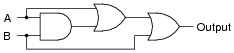• #### Question 36

Use Boolean algebra to simplify the following logic gate circuit:• #### Question 37

Use Boolean algebra to simplify the following logic gate circuit:• #### Question 38

Use Boolean algebra to simplify the following relay (ladder logic) circuit:• #### Question 39

Use Boolean algebra to simplify the following relay (ladder logic) circuit:• #### Question 40

Use Boolean algebra to simplify the following relay (ladder logic) circuit:• #### Question 41

Use Boolean algebra to simplify the following relay (ladder logic) circuit:• #### Question 42

Complete truth tables for the following gates, and also write the Boolean expression for each gate:The results should be obvious once the truth tables are both complete. Is there a general principle at work here? Do you think we would obtain similar results with Negative-OR and NAND gates? Explain.

• #### Question 43

Often, we find extended complementation “bars” in Boolean expressions. A simple example is shown here, where a long bar extends over the Boolean expression $$A + B$$:

$$\overline{A+B}$$

In this particular case, the expression represents the functionality of a NOR gate. Many times in the manipulation of Boolean expressions, it is good to be able to know how to eliminate such long bars. We can’t just get rid of the bar, though. There are specific rules to follow for “breaking” long bars into smaller bars in Boolean expressions.

What other type of logic gate has the same functionality (the same truth table) as a NOR gate, and what is its equivalent Boolean expression? The answer to this question will demonstrate what rule(s) we need to follow when we “break” a long complementation bar in a Boolean expression.

Another example we could use for learning how to “break bars” in Boolean algebra is that of the NAND gate:

$$\overline{AB}$$

What other type of logic gate has the same functionality (the same truth table) as a NAND gate, and what is its equivalent Boolean expression? The answer to this question will likewise demonstrate what rule(s) we need to follow when we “break” a long complementation bar in a Boolean expression.

• #### Question 44

What is DeMorgan’s Theorem?

• #### Question 45

Use DeMorgan’s Theorem, as well as any other applicable rules of Boolean algebra, to simplify the following expression so there are no more complementation bars extending over multiple variables:

$$\overline{\overline{AB}+\overline{AC}}$$

• #### Question 46

Use DeMorgan’s Theorem, as well as any other applicable rules of Boolean algebra, to simplify the following expression so there are no more complementation bars extending over multiple variables:

$$\overline{\overline{XY\overline{Z}}Y}$$

• #### Question 47

Use DeMorgan’s Theorem, as well as any other applicable rules of Boolean algebra, to simplify the following expression so there are no more complementation bars extending over multiple variables:

$$\overline{\overline{J+K}JL}$$

• #### Question 48

Use Boolean algebra to simplify the following logic gate circuit: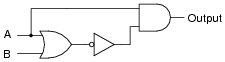• #### Question 49

Write the Boolean expression for this relay logic circuit, then reduce that expression to its simplest form using any applicable Boolean laws and theorems. Finally, draw a new relay circuit based on the simplified Boolean expression that performs the exact same logic function.• #### Question 50

Write the Boolean expression for this TTL logic gate circuit, then reduce that expression to its simplest form using any applicable Boolean laws and theorems. Finally, draw a new gate circuit diagram based on the simplified Boolean expression, that performs the exact same logic function.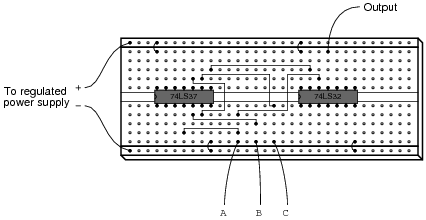• #### Question 51

A student makes a mistake somewhere in the process of simplifying the Boolean expression $$\overline{\overline{X}Y+Z}$$. Determine what the mistake is:

$$\overline{\overline{X}Y+Z}$$

$$\overline{\overline{X}Y}\overline{Z}$$

$$\overline{\overline{X}}+\overline{Y} \ \overline{Z}$$

$$X+\overline{Y} \ \overline{Z}$$

• #### Question 52

Write the Boolean expression for this TTL logic gate circuit, then reduce that expression to its simplest form using any applicable Boolean laws and theorems. Finally, draw a new gate circuit diagram based on the simplified Boolean expression that performs the exact same logic function.• #### Question 53

Suppose you needed an inverter gate in a logic circuit, but none were available. You do, however, have a spare (unused) NAND gate in one of the integrated circuits. Show how you would connect a NAND gate to function as an inverter.

Use Boolean algebra to show that your solution is valid.

• #### Question 54

Suppose you needed an inverter gate in a logic circuit, but none were available. You do, however, have a spare (unused) NOR gate in one of the integrated circuits. Show how you would connect a NOR gate to function as an inverter.

Use Boolean algebra to show that your solution is valid.

• #### Question 55

The equivalence between NAND gates and Negative-OR gates is something easily verified by an examination of these two gates’ respective truth tables, and is often a starting-point for learning about DeMorgan’s Theorem: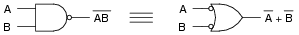A lesser-known fact is how the equivalence between NAND and Negative-OR gates may be transformed to express an equivalence between two other types of gates, shown here: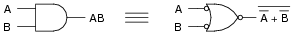Another example is shown here: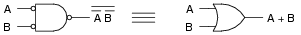Explain how the first equivalence (between the NAND and the Negative-OR gate) was transformed into the latter two equivalences, both in terms of the gate symbols and their respective Boolean expressions. In other words, explain how we can derive the last two examples by manipulating the first example.

• #### Question 56

Suppose we wished to have an AND gate for some logic purpose, but did not have any AND gates on hand. Instead, we only had NOR gates in our parts collection. Draw a diagram whereby multiple NOR gates are connected together to form an AND gate.

• #### Question 57

NAND and NOR gates both have the interesting property of universality. That is, it is possible to create any logic function at all, using nothing but multiple gates of either type. The key to doing this is DeMorgan’s Theorem, because it shows us how properly applied inversion is able to convert between the two fundamental logic gate types (from AND to OR, and visa-versa).

Using this principle, convert the following gate circuit diagram into one built exclusively of NAND gates (no Boolean simplification, please). Then, do the same using nothing but NOR gates: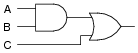• #### Question 58

An Exclusive-OR gate has the following Boolean expression:

$$A\overline{B} + \overline{A}B$$

Draw the schematic diagram for a gate circuit exhibiting this Boolean function, constructed entirely from NAND gates.

• #### Question 59

An automobile manufacturer needs a logic circuit to perform a specific task in its new line of cars. These cars will be equipped with a “headlight left on” alarm that sounds any time these two conditions are met: headlights on and ignition switch off. Draw the schematic diagram of a logic gate circuit that will implement this alarm, constructed entirely out of NAND gates.

• #### Question 60

Draw a schematic for a logic gate circuit using nothing but two-input NOR gates that mimics the operation of this relay circuit:• #### Question 61

Shown here is the ladder logic diagram for a fire alarm system, where the activation of any alarm switch opens that (normally-closed) switch contact and sounds the alarm:Write the Boolean expression for this relay circuit, then simplify that expression using DeMorgan’s Theorem and draw a new relay circuit implementing the simplified expression.

• #### Question 62

The Law of Distribution in boolean algebra is identical to the law of distribution in “normal” algebra:

$$A(B + C) = AB + AC \ \ \ \ \ \ \ \ \ \ \ \ \ Applying \ the \ Law \ of \ Distribution$$

While the process of distribution is not difficult to understand, the reverse of distribution (called factoring) seems to be a more difficult process for many students to master:

$$AB + AC = A(B + C) \ \ \ \ \ \ \ \ \ \ \ \ \ Factoring \ A \ out \ of \ each \ term$$

Survey the following examples of factoring, and then describe what this process entails. What pattern(s) are you looking for when trying to factor a Boolean expression?

$$CD + AD + BD = D(C + A + B)$$

$$X\overline{Y} \ \overline{Z}+\overline{X} \ \overline{Y} Z = \overline{Y}(X\overline{Z}+\overline{X}Z)$$

$$J + JK = J(1 + K)$$

$$AB + ABCD + BCD + B = B(A + ACD + CD + 1)$$

• #### Question 63

Simplify this logic gate circuit, which uses nothing but NAND gates to accomplish a certain logic function:• #### Question 64

Simplify this logic gate circuit, which uses nothing but NOR gates to accomplish a certain logic function:• #### Question 65

Sum-of-Products (SOP) expressions may be implemented by a combination of AND and OR gates, as such:Use DeMorgan’s Theorem to prove that this NAND gate circuit performs the exact same function:• #### Question 66

Write the Boolean expression for this logic gate circuit, then reduce that expression to its simplest form using any applicable Boolean laws and theorems. Finally, draw a new gate circuit diagram based on the simplified Boolean expression that performs the exact same logic function.• #### Question 67

Write the Boolean expression for this logic gate circuit, then reduce that expression to its simplest form using any applicable Boolean laws and theorems. Finally, draw a new gate circuit diagram based on the simplified Boolean expression that performs the exact same logic function.